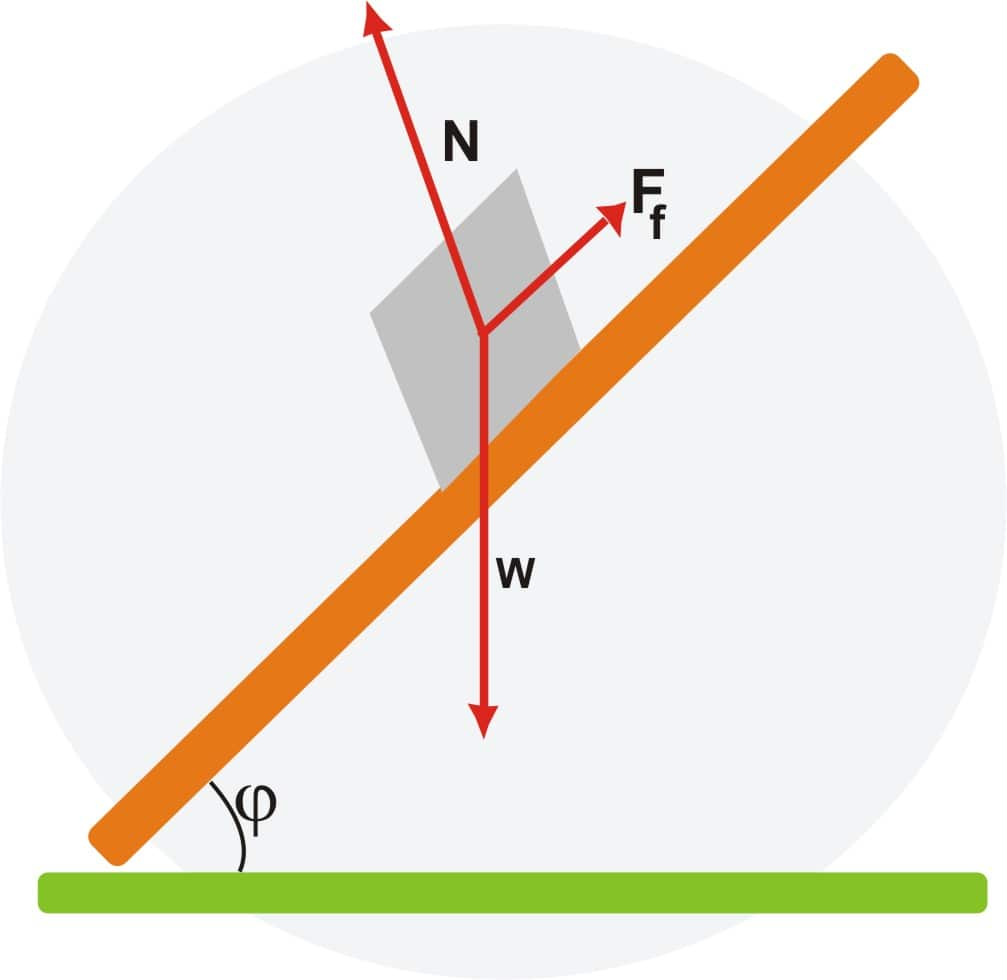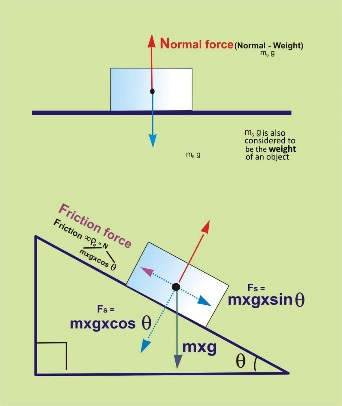# Sliding Friction

## What is Sliding Friction - Definition and Examples

We can understand sliding friction as the resistance force created between any two bodies when sliding against each other. This friction is also called kinetic friction and it is defined as the force that is required to keep a surface sliding along another surface. It hangs on two variable- one is material or the thing and other is the weight and size of the object. Any variation in the surface area and in contact does not change the sliding friction. In most of the materials, sliding friction is much less than the static friction.Sliding can occur among two objects of random shape, whereas rolling friction is the frictional force related to the rotational movement of a somewhat disc-like or any other circular object along a surface. Usually, the frictional force of rolling friction is much less than that related to sliding kinetic friction. Usual values for the coefficient of rolling friction are smaller than that of sliding friction. Similarly, sliding friction usually produces more sound and more thermal bi-products. One example is braking motor vehicle tires on a roadway, a process which generates considerable heat and sound, and is taken into reason in measuring the magnitude of roadway noise pollution. We can take a simple example is when we stop our car at stop sign then it slows down due to friction between applied breaks and the wheels. Thus, the force which is acted in the opposite direction where a body wants to slide is called sliding friction Some key categories of friction are rolling, sliding, static, fluid friction. Here we will discuss sliding friction or kinetic friction, its coefficient through which it is measured, and its examples.

Sliding Friction Definition

The frictional force which resists the real relative sliding motion between two contact surfaces is known as sliding or kinetic friction. Let us begin with studying frictional forces from simple understanding. Suppose there is a metal block on a table, a weak force may not set the metal block into motion. As you keep increasing the force progressively, with a particular amount of force, the metal block starts moving. The controlling value of the force at which the metal block starts moving is the same as the resistive force offered by the metal block under the static form. Hence that resistive force is called Static Friction. Ongoing the experiment, increasing the force further, it makes the metal block move. But even after the metal block started moving, it still provides a resistive force trying to oppose the motion. It is defined as the ‘sliding friction’. From what we termed so far, it is clear that the sliding friction is smaller than the static friction.

The force of sliding friction is directly proportional to the weight, acting in the direction normal to the surface. As a specific case, if the surface on which the body slides is horizontal, then the normal force matches the weight of the object.Sliding Friction Formula

The equation for sliding force contains the coefficient of sliding friction times the normal force.
FS = μSFn

F_{s}=\mu_{s}F_{n}

Where,
FS F_{s} = force of sliding friction
μS \mu_{s} = Coefficient of sliding friction
Fn  F_{n} = normal force
Motion under Sliding Friction.
The motion under sliding friction can be showed (in simple systems of motion) by Newton's Second Law
∑ F= ma \sum F= ma
FE – FK = ma F_{E}-F_{K}=ma
Where FE is an external force.
·         Acceleration happens when the outside force is greater than the force of kinetic friction.
·         Slowing Down (or Stopping) happens when the force of kinetic friction is bigger than that of the outside force.
·         This is also followed by Newton's first law of motion as there exists a net force on the object.
• • Constant Velocity happens when there is no net force on the object, that is the outside force is equal to the force of kinetic friction

• Motion on an inclined plain

A block can be used to understand friction as it slides up or down an inclined plane. This is shown in the free body diagram belowThe component of the force of gravity in the direction of the incline is shown by:
Fg = mg sinᶿ F_{g}=mg sin^{\theta}

The normal force (perpendicular to the surface) is shown by.
N= mg cosᶿ N=mg cos^{\theta}

Therefore, since the force of friction resists the motion of the block
Fk= µk · mg cosᶿ F_{k}=\mu_{k}. mg cos^{\theta}

To find the coefficient of kinetic friction on an inclined plane, one must see that the moment where the force is parallel to the plane is same to the force perpendicular; this occurs when the object is moving at a constant velocity at some angle {\displaystyle \theta }

{\displaystyle F_{g}=mg\sin {\theta }}
∑ F = ma =0 \sum F=ma=0
Fk = Fg or = µk · mg cosᶿ = mgcosᶿ
F_{k}=F_{g} or \mu_{k}.mg cos^{\theta}=mgcos^{\theta}

Understanding Sliding Friction

Sliding friction produced or generated by objects is said as a coefficient which taken into consideration the several factors that can affect the level of friction. These several factors that can impact sliding friction include the following:

• • The surface distortion of objects.

• • The roughness or smoothness of the surface.

• • The original velocity of either object.

• • The size and shape of the object.

• • The amount of pressure on any object.

• • The adhesion force of the surface.

• Sliding Friction Characteristics

We have already explained before that in general, sliding friction is always smaller than static friction for the same set of the body and the surface movement. This also leads to another conclusion that the frictional force always depends on the nature of the material of the object and surface. As also explained in past a sliding force is proportional to the normal force, which means the load of the object. In the experimentation, we had clarified in the previous section the amount of the sliding friction will be equal even when you change the side of the block that rests on the table. Hence, for the equal mass, the sliding friction is free of the area of contact. The sliding friction is also independent of the speed of motion.

The coefficient of Sliding Friction

We have given an overall definition that a frictional force F seen by an object is directly proportional to the normal force N exerted by that. That is,
F=μNF=μN F=\mu NF=\mu N

Where µ is a constant it is called coefficient of friction. It is clearly a ratio of two forces and therefore it has no dimensions. If the friction is static, then the coefficient of friction is named as ‘coefficient of static friction’ and denoted by μs and for sliding friction the same constant is known as ‘coefficient of sliding friction’ and is denoted by μk. The subscript ‘k’ is used to mean as ‘kinetic’. Then sliding friction is always smaller than static friction and so,  μk < μs.

Sliding Friction Examples

There are not various examples of sliding friction as the degree of sliding friction is extensive. The sliding friction between two surfaces produces heat due to molecular interactions. The amount of heat produced depends on the materials of the surfaces and might turn into a fire in sometimes. Lighting a match stick is an extraordinary example in present-day life. Stone age men used two stones for ignition.  Because of a high degree of frictional force in sliding friction, one favors to put an object on wheel and transport instead of pushing along because of the fact that result of rolling friction is far smaller than that of sliding friction. The values for the coefficient of rolling friction are quite small than that of sliding friction.

Imagine a car parked on an inclined plane with a less gradient. In the absence of sliding friction, the wheels of the car will begin to rotate and start moving. This is the reason, at steep gradients, hand brakes are used while parking and in worst cases, heavy stones are placed behind the tires. Sliding friction, because of a considerable level, in a way is helpful in real life.

A few examples like for sliding friction

• • Pushing a heavy and bigger object such as crowbar

• • Ribbing a weight on ramps

• • Movement of coins in a carom board can be cited